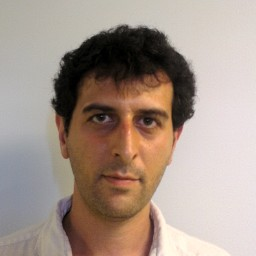# Team : PolSys - Polynomial Systems

Axes : SSR (👥👥), TMC (👥👥).

Mohab Safey El Din Campus Pierre et Marie Curie 26-00/321

## Short presentation

The PolSys team activity is centered on the design of algorithms based on computer algebra and symbolic computation to solve systems of polynomial constraints, the implementation of these algorithms and their applications.
The fundamental problem of solving systems of polynomial constraints arises in a wide range of areas such as cryptology, computational geometry, program verification in computer science, mathematical conjectures of geometric or combinatorial nature, and in engineering sciences such as robotics, biology, chemistry to cite a few.
The nonlinear nature of these problems and the requirements on exactness and exhaustiveness which arise in some applications make the use of local numerical and approximation methods difficult. Hence, the algorithmic challenges for solving polynomial systems are numerous, especially when considering that this problem is NP-hard. By using methods from computer algebra such as Gröbner bases computations, the PolSys team designs algorithms which allow:

• the solving of polynomial systems with coefficients in a finite field (for applications in e.g. cryptology or error correcting codes) or with exact rational coefficients (for applications in engineering sciences)
• the solving over the reals of systems of polynomial constraints, even when the number of complex solutions is infinite, or depending on parameters (for applications of geometric nature or coming from robotics)
• the solving of quantifier elimination problems over the real or the complex numbers and polynomial optimization problems (for applications in program verification or coming from engineering sciences)
• the determination of topological properties (such as number of connected components) of real solution sets to systems of polynomial constraints.
In order to solve this wide range of algorithmic problems, the PolSys team develops several dedicated software. In particular, the msolve C library is already used in several computer algebra systems such as SageMath and OSCAR (see also other software developed by the team).
Also, the team has a regular activity in several application areas such as cryptology and robotics.

Staff directory

Computer Algebra. Polynomial System Solving. Gröbner Bases. Complexity. Real roots. Parametric systems. Cryptology. Algebraic Cryptanalysis. Algebraic Computational Geometry. Applications. Symbolic/Numeric Interaction. Software. High performance Linear Algebra.

http://www-polsys.lip6.fr

No event planned at present.

Archives

All

## Contact

mohab.safey (at) nulllip6.fr